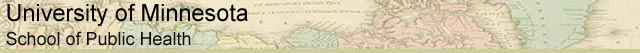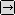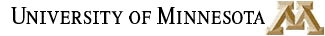### MATH REVIEW: USEFUL MATH FOR EVERYONE

SECTION 3. WHAT IS AN EXPONENT?

An exponent refers to the number of times a number is multiplied by itself. For example, 2 to the 3rd (written like this: 23) means:

2 x 2 x 2 = 8.

23 is not the same as 2 x 3 = 6.

Remember that a number raised to the power of 1 is itself. For example,

a1 = a

51 = 5.

There are some special cases:

1. a0 = 1

When an exponent is zero, as in 60, the expression is always equal to 1.

a0 = 1

60 = 1

14,3560 = 1

2. a-m = 1 / am

When an exponent is a negative number, the result is always a fraction. Fractions consist of a numerator over a denominator. In this instance, the numerator is always 1. To find the denominator, pretend that the negative exponent is positive, and raise the number to that power, like this:

a-m = 1 / am

6-3 = 1 / 63

You can have a variable to a given power, such as a3, which would mean a x a x a. You can also have a number to a variable power, such as 2m, which would mean 2 multiplied by itself m times. We will deal with that in a little while.to Exponents, Page 2